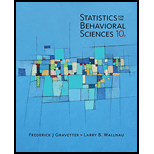# A researcher interested in the texting habits of high school students in the United States. The researcher selects a group, of 100 students, measures the number of text message that each individual sends each day, and calculate. the average number for the group. Identify the population for this study. Identify the sample for this study. The average number that the researcher calculated is, an example of a ________.### Statistics for The Behavioral Scie...

10th Edition
Frederick J Gravetter + 1 other
Publisher: Cengage Learning
ISBN: 9781305504912### Statistics for The Behavioral Scie...

10th Edition
Frederick J Gravetter + 1 other
Publisher: Cengage Learning
ISBN: 9781305504912

#### Solutions

Chapter
Section
Chapter 1, Problem 1P
Textbook Problem

## A researcher interested in the texting habits of high school students in the United States. The researcher selects a group, of 100 students, measures the number of text message that each individual sends each day, and calculate. the average number for the group.Identify the population for this study.Identify the sample for this study.The average number that the researcher calculated is, an example of a ________.

Expert Solution
To determine

Identify population and sample for the study,also determine if the average calculated by the researcher is a parameter or statistic.

Solution:

a) Population is high school student in United States.

b) Sample is group of 100 high school students.

c) The average number that the researcher calculated is an example of Statistic

### Explanation of Solution

Population is the entire set of the individuals of interest for a particular research question.

A Sample is a set of individuals selected from a population usually intended to represent the population in a research study.

Statistic is a value usually a numerical value that describes a sample. A statistic is usually derived from measurements of the individuals in the sample.

### Want to see more full solutions like this?

Subscribe now to access step-by-step solutions to millions of textbook problems written by subject matter experts!

Get Solutions

### Want to see more full solutions like this?

Subscribe now to access step-by-step solutions to millions of textbook problems written by subject matter experts!

Get Solutions

Find more solutions based on key concepts
Show solutions
A sample of n = 9 scores has X = 108. What is the sample mean?

Essentials of Statistics for The Behavioral Sciences (MindTap Course List)

Solve the equations in Exercises 112 for x (mentally, if possible). 7x+55=98

Finite Mathematics and Applied Calculus (MindTap Course List)

71-82 Evaluate the integral. 1ex2+x+1xdx

Calculus (MindTap Course List)

Factor completely: a. 12x3 10x2 12x b. 2bx 2by + 3cx 3cy

Applied Calculus for the Managerial, Life, and Social Sciences: A Brief Approach

Evaluate the integrals in Problems 1-32. Identify the formula used. 16.

Mathematical Applications for the Management, Life, and Social Sciences

Explain why there is no angle such that sin = 2.

Trigonometry (MindTap Course List)

True or False: If f(x) 0 for all x in an interval I then f is increasing on I.

Study Guide for Stewart's Single Variable Calculus: Early Transcendentals, 8th

Find all real solutions of each equation. 5a2=a+6

College Algebra (MindTap Course List)

40. The prior probabilities for events A1, A2, and A3 are P(A1) = .20, P(A2) = .50, and P(A3) = .30. The condit...

Modern Business Statistics with Microsoft Office Excel (with XLSTAT Education Edition Printed Access Card) (MindTap Course List)

In Problems 2730 express the solution of the given initial-value problem in terms of an integral-defined functi...

A First Course in Differential Equations with Modeling Applications (MindTap Course List)

Reminder Round all answers to two decimal places unless otherwise indicated. Wages A worker is reviewing his pa...

Functions and Change: A Modeling Approach to College Algebra (MindTap Course List)